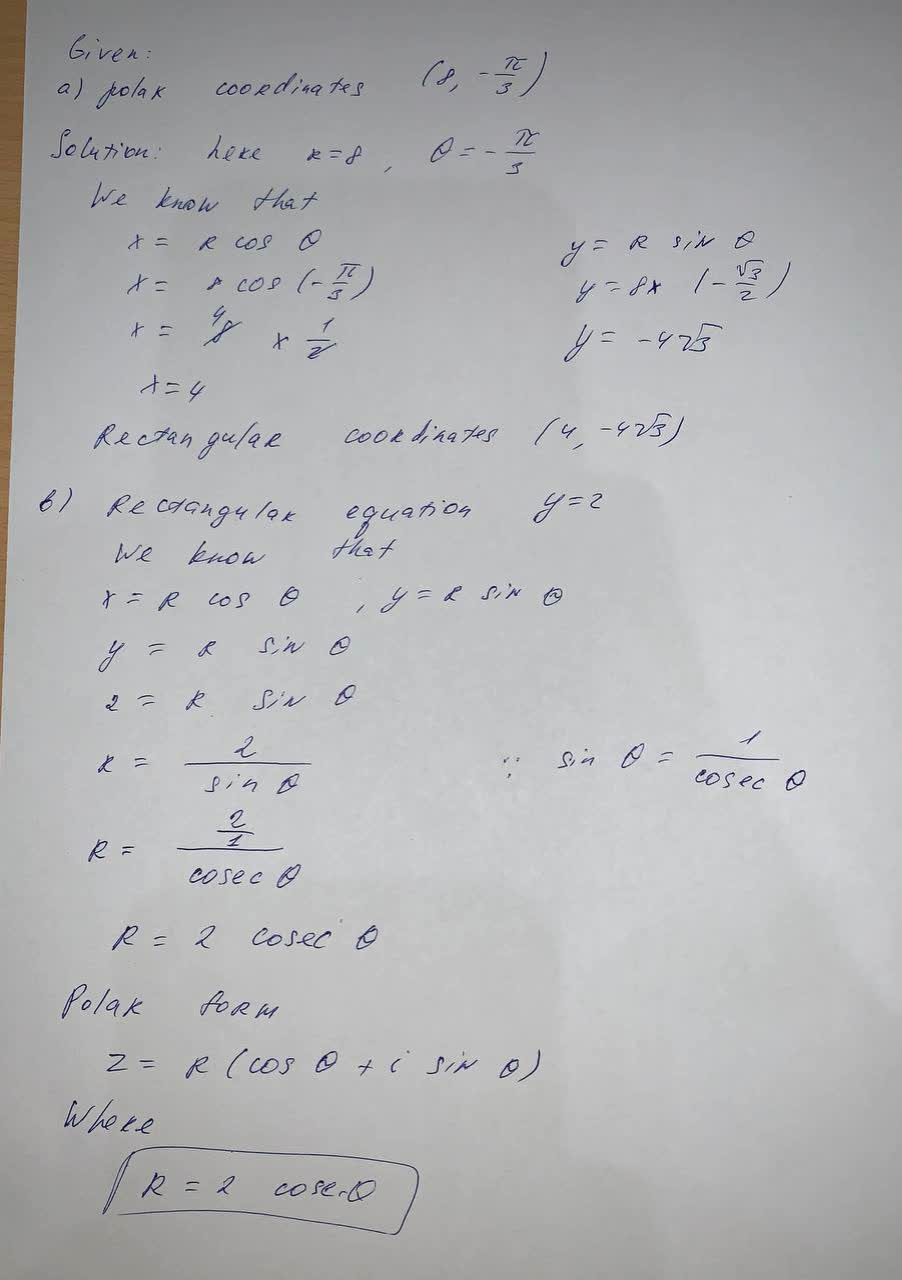Rectangular equation y=2 convert to polar equationCheyanne Leigh 2021-09-07 Answered
Rectangular equation y=2 convert to polar equation

• Questions are typically answered in as fast as 30 minutes

Solve your problem for the price of one coffee

• Math expert for every subject
• Pay only if we can solve itkrolaniaN

The answer is given below in the photo